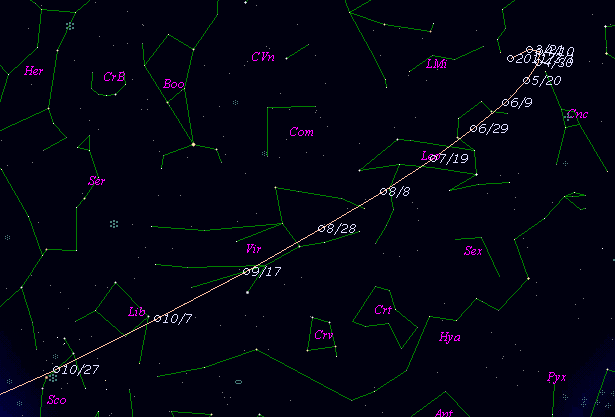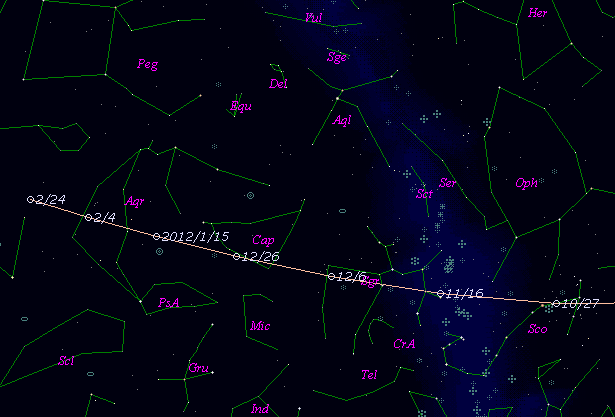# 73P/Schwassmann-Wachmann 3 (2011)###Pictures###Orbital Elements

```Epoch 2011 Oct. 6.0 TT = JDT 2455840.5
T 2011 Oct. 16.89347 TT                                 MPC
q   0.9427968            (2000.0)            P               Q
n   0.18382640     Peri.  198.86377     -0.02850687     +0.98228417
a   3.0633560      Node    69.84463     -0.88986542     +0.05946540
e   0.6922340      Incl.   11.37904     -0.45533163     -0.17771233
P   5.36
From 2786 observations 2000 Nov. 7-2010 Dec. 17, mean residual 0"..
Nongravitational parameters A1 = +0.76, A2 = +0.0258.
```

###Finding Charts###Magnitudes Graph

```	Component C:
m1 =  9.7 + 5 log d + 16.5 log r  [   ,-76]  (             - 2011 Aug.  1)
m1 = 11.2 + 5 log d +  6.5 log r  [-76,  0]  (2011 Aug.  1 - 2011 Oct. 16)
m1 = 11.4 + 5 log d + 15.0 log r  [  0,   ]  (2011 Oct. 16 -             )

Component B:
m1 = 12.0 + 5 log d + 20.0 log r(t + 30)
```##### The orbital elements are published on M.P.E.C. 2010-Y12. The charts are made with StellaNavigator Ver.2.0 for Windows (AstroArts / ASCII). The magnitudes graphs are made with Comet for Windows.